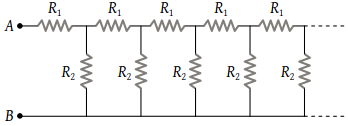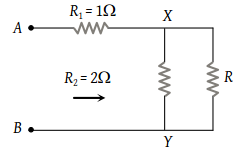# NEET Physics Current Electricity Questions SolvedAn infinite sequence of resistance is shown in the figure. The resultant resistance between A and B will be, when R1 = 1 ohm and R2 = 2 ohm(1) Infinity

(2) 1 Ω

(3) 2 Ω

(4) 1.5 Ω

(3) Let the resultant resistance be R. If we add one more branch, then the resultant resistance would be the same because this is an infinite sequence.$\therefore \frac{R{R}_{2}}{R+{R}_{2}}+{R}_{1}=R⇒2R+R+2={R}^{2}+2R$

$⇒{R}^{2}-R-2=0⇒R=-1$ or $R=2\text{\hspace{0.17em}}ohm$

Difficulty Level:

• 18%
• 22%
• 45%
• 18%
Crack NEET with Online Course - Free Trial (Offer Valid Till September 21, 2019)